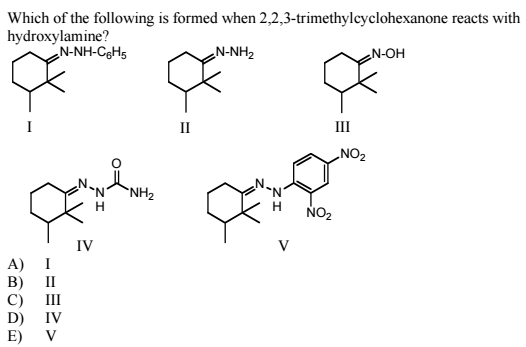Problem: Determine the correct answer for the question below.

87% (11 ratings)
Problem Details

Determine the correct answer for the question below.What scientific concept do you need to know in order to solve this problem?

Our tutors have indicated that to solve this problem you will need to apply the Addition of Amine Derivatives concept. You can view video lessons to learn Addition of Amine Derivatives Or if you need more Addition of Amine Derivatives practice, you can also practice Addition of Amine Derivatives practice problems .

What is the difficulty of this problem?

Our tutors rated the difficulty of Determine the correct answer for the question below. ... as medium difficulty.

How long does this problem take to solve?

Our expert Organic tutor, Chris took 2 minutes to solve this problem. You can follow their steps in the video explanation above.

What professor is this problem relevant for?

Based on our data, we think this problem is relevant for Professor Daoudi's class at UCF.